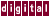# 7.5 Loop Rerolling

Many codes have loops that were unrolled manually over several iterations to amortize the cost of the test and branch at each iteration of the `for` loop. Before KAP can examine them, they must be rerolled to a simpler form, as shown in the following code examples:

```for (i=0; i<n; i+=2) {
a[i] = b[i]+c[i];
a[i+1] = b[i+1]+c[i+1];
}
```

KAP recognizes the following code as an unrolled loop and rerolls it before applying loop-based optimizations:

```for ( i = 0; i<=(n + 1) / 2 * 2 - 1; i++ ) {
a[i] = b[i] + c[i];
}
```

Unrolled summations are also recognized, as follows:

```sum = 0.0;
for ( i=0; i<n; i+=4 ) {
sum += b[i];
sum += b[i+1];
sum += b[i+2];
sum += b[i+3];
}
```

Becomes:

```sum = (float )(0.0);
for ( i = 0; i<=(n + 3) / 4 * 4 - 1; i++ ) {
sum +=  b[i];
}
```

Previous Page Next Page Contents Index
Command-Line Qualifiers